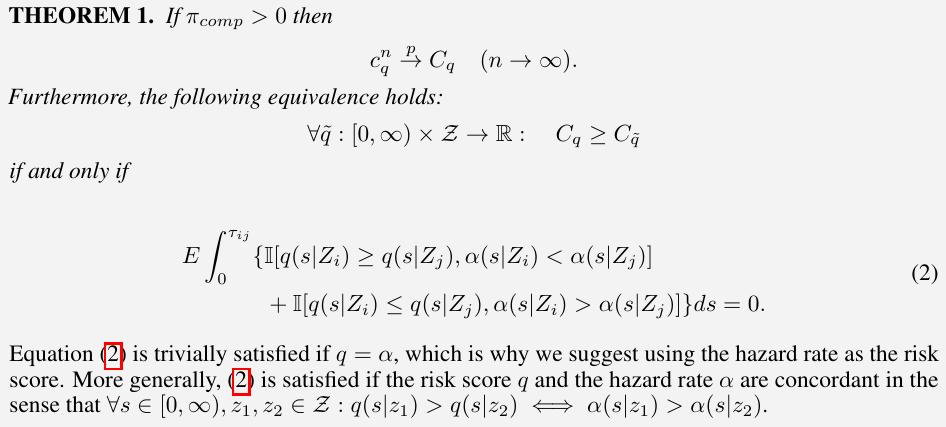# C-index for Time-varying Risk

##### Posted on May 05, 2023
Tags: Cox Model, C-index, Time-varying

Harrel’s C-index is used for the risk of individuals is time-dependent, such as the proportional hazards model.

The paper shows that

in the limit, concordance is maximized iff the risk score is concordant with the hazard rate, in the sense that for a comparable pair where the first event time is observed, the risk score is concordant with the hazard rate at the first event time.

Thus, the paper suggested using the hazard rate as the risk score when calculating concordance.

• by simulations, they demonstrate situations where other concordance indices can lead to incorrect models being selected over a true model, justifying the use of our suggested risk prediction in both model selection and in loss functions.

## Introduction

C-index is first developed as an adaptation of the Kendall-Goodman-Kruskal-Somers type rank correlation index to right-censored survival data, similar to an adaptation of Kendall’s $\tau$.

• $(X_i, U_i, Z_i), i\in \bbN$ be iid with the lifetime $X_i$ and the right censoring time $U_i$ being non-negative random variables. Let $Z_i$ be an element of some space.
• observe $(T_i, D_i, Z_i), i=1,\ldots, n$, where $T_i=\min(X_i, U_i)$ is the time at risk and $D_i = \bbI(X_i\le U_i)$ is the event indicator

The pair is comparable when the first event is not a censoring event. The probability that individual $i$ has such an event occurring before event $j$ is

$\pi_{comp} = P(D_i=1, T_i < T_j)$

For risk score that depends on the covariate and on time, we compare the risk scores at the time when the first event occurs.

Formally, the risk score is specified through a function $q:[0, \infty)\time Z\rightarrow \bbR$ and for a given pair $(i, j)$, with $T_i < T_j$, we say that $i$ has a higher risk score than $j$ if $q(T_i\mid Z_i) > q(T_i\mid Z_j)$.

Higher values of the risk score indicate a propensity towards earlier events.

The probability of a pair having observed the event of $i$ before the event of $j$ and being concordant is

$P[D_i=1, T_i < T_J, q(T_i\mid Z_i) >q(T_i\mid Z_j)]\,.$

Divide the above by $\pi_{comp}$:

• a perfect model that could correctly order every pair would have a concordance of 1
• a model that simply guesses for each pair would have a concordance of 0.5 on average

In the C-index

$C_q = \frac{\pi_{conc}}{\pi_{comp}}$

we use

$\pi_{conc} = P[D_i=1, T_i< T_j, q(T_i\mid Z_i) > q(T_i\mid Z_j)] +\frac 12P[D_i=1, T_i< T_j, q(T_i\mid Z_i)=q(T_i\mid Z_j)]$

denote the estimated C-index as

$c_q^n = \hat\pi_{conc} / \hat\pi_{comp}$

Often, the risk score $q(t\mid z)$ being used is not dependent on the first argument $t$, i.e., $q(t\mid z) = z\hat\beta$.

For more general survival models, where we have access to a survival function $S(t\mid z)$ as a function of the covariate $z$, a definition of a risk score is less obvious, as

there may not be a clear definition of what constitutes higher risk, for example when the underlying hazard rates of individual cross.

several methods:

• $q(t\mid Z) = -S(t_0\mid Z)$: negative of the survival function evaluated at some fixed time $t_0 > 0$
• $q(t\mid Z) = -\inf{t\,\text{s.t.}\,S(t\mid Z) \le 0.5}$

These suggestions does not depend on the first argument of $q$.

Antolini et al. (2015): introduce a time-dependent concordance index. This adaptation of the C-index is developed for models with either time-varying covariates or time-varying effects, while supposing the predicted survival function is the “natural” relative risk predictor.

It leads to an event-time dependent risk score

$q(t\mid Z) = -S(t\mid Z)$

The index is used widely in deep learning survival models, see

• Zhong et al. (2021)
• Lee et al. (2018)

The paper suggest using the conditional hazard rate $q(t\mid z) = \alpha(t\mid z)$Published in categories Note